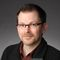cancel
Showing results for
Did you mean:
Collaborate forum
cancel
Showing results for
Did you mean:

## wall thickness geometry .ifc definition raw data analysis of coordinatesAnonymous
Not applicable
Hello,

I am trying to analyze results from the raw .ifc file I exported which describe the geometry of a wall layer thickness. In this case the layer thickness should be 10mm. The data follows the IFC definition in the following link:

http://www.buildingsmart-tech.org/ifc/IFC4/final/html/schema/ifcgeometricmodelresource/lexical/ifctriangulatedfaceset.htm

From the attached image you can see the example data that is given by the raw .ifc file in terms of:

1) coordinate pairs (x,y,z)
2) indexes in the form of (0.,-0.707106781187,-0.707106781187)
3) indexes in the form of (1,4,2), these I understand pertain to the nodes composing the triangulation
4) indexes in the form of (2,2,2), these describe a straight line (from (1,1,1,) to (84,84,84,)

My questions are:

- What is the relationship between 1) and 3) exactly? there are 164 coordinates and twice as many indexes of triangulation so the mathematical or geometrical relation isn't very evident.

- It isn't clear what 2) represents... are these some parameterized values?

- And finally, what does 4) represent? A straight line across the geometry from 'startpoint' to 'endpoint' ?

Thank you
2 REPLIES 2Booster
The first list of triplets (not pairs) contains X,Y,Z position coordinates for the vertices. The second list seems to contain normal vectors (X,Y,Z) (per vector?).

The others will be the indices to define the actual triangles.
I don't think you'll find UV Texture Coordinates (pairs of U,V values).
--- stefan boeykens --- architect-engineer-musician ---
MBP2019:i9Octo2.4GHz32GBVega20/BigSur+Win11Anonymous
Not applicable
Hi,
I'm not trying to find texture U,V...

I know that the coordinate triplets are vertices locations and the indices are for the triangles... this is explained in the link I mentioned for the actual definition of the dataset...

What I'm looking for is the relation BETWEEN the coordinates and the indices.Latest solutions

Most liked posts